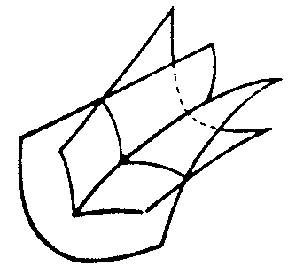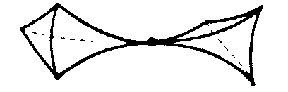# Thom catastrophes

Singularities of differentiable mappings, whose classification was announced by R. Thom  in terms of their gradient dynamical systems and the analogous list of critical points of codimension $\leq 4$( cf. Critical point) of differentiable functions. The original formulation of Thom's result is that a generic four-parameter family of functions is stable, and in the neighbourhood of a critical point it behaves, up to sign and change of variable, like one of seven cases (cf. Table).

<tbody> </tbody>
 Notation Codim Corank Germ Universal deformation Name $A _ {2}$ 1 1 $x ^ {3} + y ^ {2}$ $ux$ Fold $A _ {3}$ 2 1 $x ^ {4} + y ^ {2}$ $ux + vx ^ {2}$ Cusp $A _ {4}$ 3 1 $x ^ {5} + y ^ {2}$ $ux + vx ^ {2} + ux ^ {3}$ Swallow-tail $D _ {4} ^ {-}$ 3 2 $x ^ {3} + xy ^ {2}$ $ux + vx ^ {2} + wy$ Hyperbolic umbilic $D _ {4} ^ {+}$ 3 2 $x ^ {3-} xy ^ {2}$ $ux + vx ^ {2} + wy$ Elliptic umbilic $A _ {5}$ 4 2 $x ^ {6} + y ^ {2}$ $ux + vx ^ {2} + wx ^ {3} + tx ^ {4}$ Butterfly $D _ {5}$ 4 2 $x ^ {4} + xy ^ {2}$ $ux + vx ^ {2} + wx ^ {3} + ty$ Parabolic umbilic

The germs (cf. Germ) corresponding to the Thom catastrophes are finitely determined (specifically, $6$- determined: in appropriate coordinates they correspond to polynomials in two variables of degrees $\leq 6$).

The codimension $\mathop{\rm codim}$ serves as a measure of the complexity of a critical point. Any small perturbation of a function $f$ of $\mathop{\rm codim} r$ leads to a function with at most $r$ complex critical points. The codimension of a singularity (that is, of a germ $f$ such that $f ( 0) = Df ( 0) = 0$) is the number $\mathop{\rm dim} \mathfrak m /\langle \partial f \rangle$, where $\mathfrak m = \{ {g } : {g( 0) = 0 } \}$ and $\langle \partial f \rangle$ is the ideal generated by the germs $\partial f/ \partial x ^ {i}$. For example, if $f = x ^ {N}$, then $\langle \partial f \rangle = \langle x ^ {N - 1 } \rangle$, and the residue classes of $x \dots x ^ {N - 2 }$ form a basis of $\mathfrak m /\langle \partial f \rangle$, so that $\mathop{\rm codim} = N- 2$. The inequality $\mathop{\rm codim} f \geq c ( c + 1)/2$, holds, where $c$ is the corank of the Hessian $\partial ^ {2} f/ \partial x ^ {i} \partial x ^ {j} ( 0)$. Hence, in particular, if $r \leq 4$, then $c \leq 2$.

Finite determination (sufficiency) of a germ, roughly speaking, means that it is determined, up to smooth change of coordinates, by its jets (cf. Jet). More precisely, a germ $f$ is said to be $k$- determined if every germ $f _ {1}$ with the same $k$- jet (that is, the same Taylor series up to order $k$) as $f$ is right equivalent to $f$( i.e. $f _ {1} = f \circ \varphi$ where $\varphi$ is the germ at $0$ of a diffeomorphism; cf. ). A germ is finitely determined if and only if it has finite codimension. In particular, if $\mathop{\rm codim} = r$, then $f$ is $( r + 2)$- determined (whence $6$- determined for $r \leq 4$).

The Thom catastrophes, in contrast to the case of general position, are degenerate singularities (that is, the Hessian is degenerate at them), and they can be removed by a small perturbation, as mentioned above. However, in many cases of practical importance, and also theoretically, one is interested not in an individual object, but in a collection of them, depending on some "control" parameters. Degenerate singularities which are removable for each fixed value of the parameters may be removable for the collection as a whole. (Stability of Thom catastrophes may also be considered in this sense.) But then the natural object of study is not the singularity itself, but a collection (a deformation of the singularity) in which it is non-removable (or disintegrates, or "bifurcates" ) under a change of parameters. It turns out that in many cases the study of all possible deformations can be reduced to the study of a single one, which is in a certain sense so big that all the others can be obtained from it. Such deformations are called versal and they, in turn, can be obtained from a universal (or miniversal) deformation, which is characterized by having least possible dimension of its parameter space. The most important result here is Mather's theorem: A singularity $f$ has a universal deformation if and only if its codimension is finite.

A deformation $F ( x, u)$, $x \in \mathbf R ^ {n}$, $u \in \mathbf R ^ {r}$, of a germ $f ( x)$, $F ( x, 0) = f ( x)$, is given by a formula

$$F ( x, u) = \ f ( x) + b _ {1} u _ {1} + \dots + b _ {r} u _ {r} ,$$

where $( b _ {1} \dots b _ {r} )$ is an arbitrary collection of representative elements of a basis of the space $\mathfrak m /\langle \partial f \rangle$. Thom catastrophes correspond to deformations with at most four parameters.

Important for applications is the so-called bifurcation set, or singular set, $D _ {f} = \{ {( x, u) \in \mathbf R ^ {n} \times U } : {d _ {x} f = 0 \textrm{ and } d ^ {2} f \textrm{ is degenerate } } \}$; its projection to the $u$- space, the set $\{ {u \in U } : {( x, u) \in D _ {f} \textrm{ for some } x \in \mathbf R ^ {n} } \}$, is called the catastrophe set. It lies in the control space and hence is "observable" , and all "discontinuities" or "catastrophes" originate from it. Fig.1a, Fig.1b and Fig.1c illustrate the cases corresponding to $\mathop{\rm codim} 3$.Figure: t092650aFigure: t092650bFigure: t092650c

How to Cite This Entry:
Thom catastrophes. Encyclopedia of Mathematics. URL: http://encyclopediaofmath.org/index.php?title=Thom_catastrophes&oldid=53960
This article was adapted from an original article by M.I. Voitsekhovskii (originator), which appeared in Encyclopedia of Mathematics - ISBN 1402006098. See original article